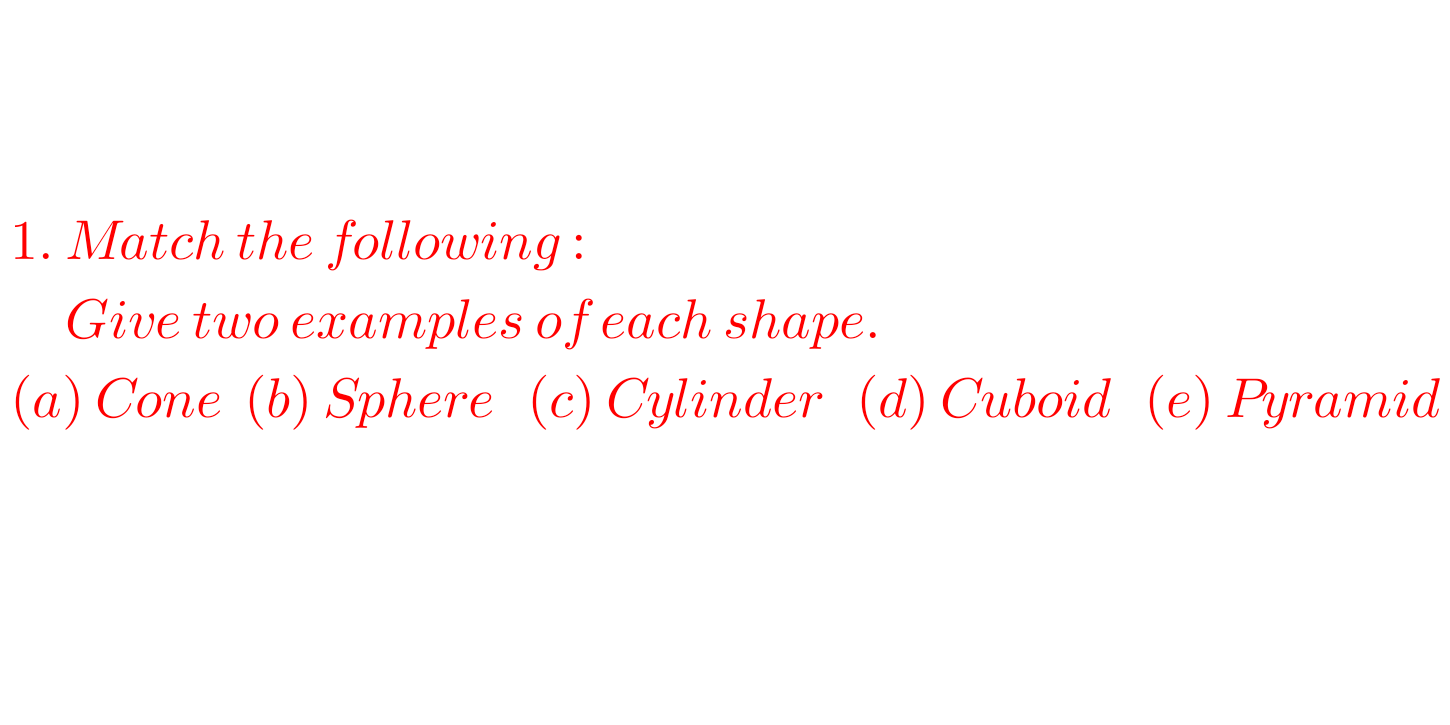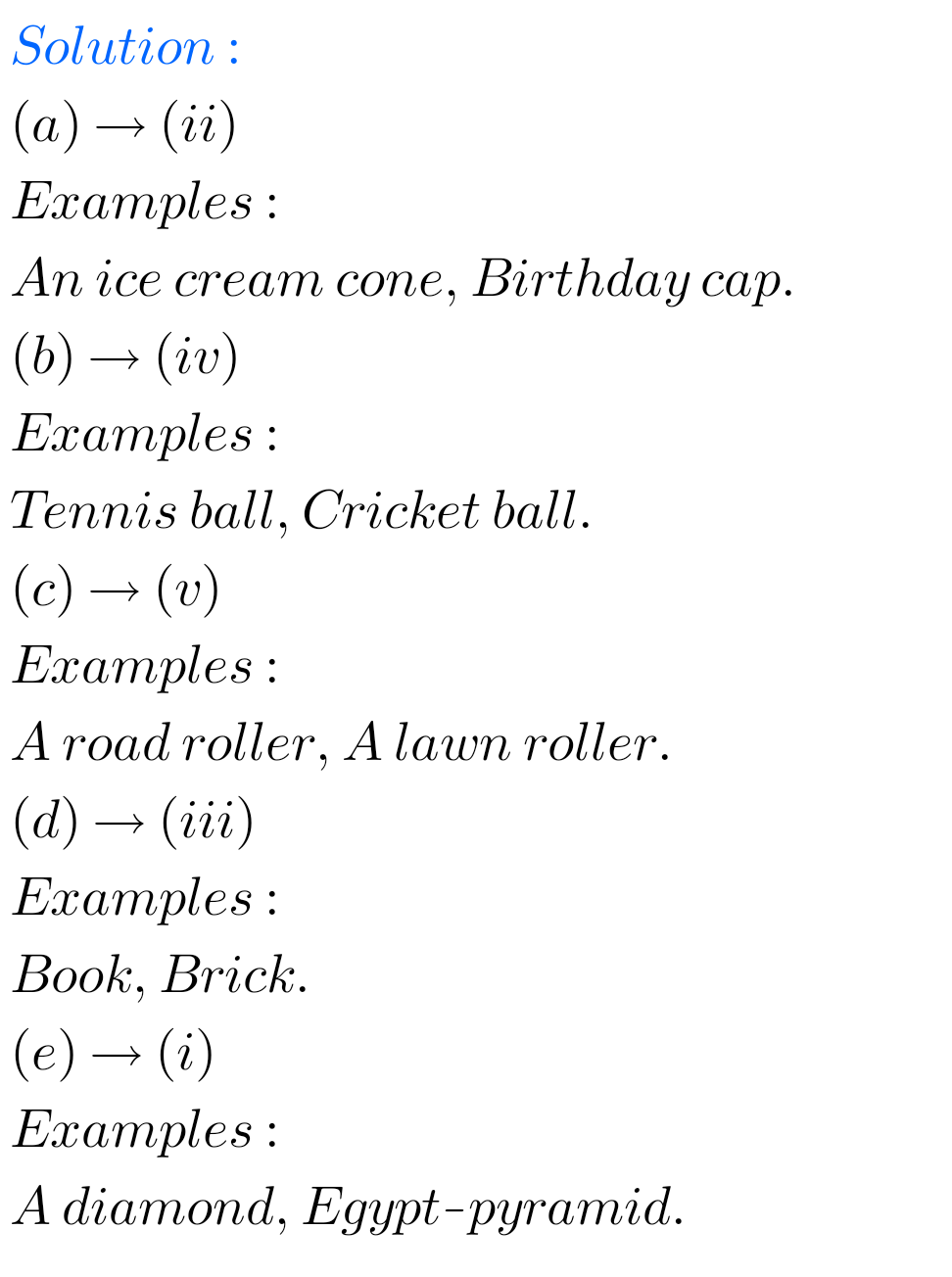# Exercise 5.9 solutions chapter 5 class 6 Maths Ncert Understanding Elementary Shapes

Ncert Maths Class 6 chapter 5 Understanding Elementary Shapes Exercise 5.9 solutions are given.

Study the textbook lesson Understanding Elementary Shapes very well.

Observe the example problems and solutions given in the textbook.

Observe the given solutons and try them in your own method.

You can see

Ncert maths solutions for class 6
Understanding elementary shapes

Exercise 5.1

Exercise 5.2

Exercise 5.3

Exercise 5.4

Exercise 5.5

Exercise 5.6

Exercise 5.7

Exercise 5.8

Exercise 5.9

M

# Exercise 5.9 chapter 5 solutions class 6 Ncert Maths

Chapter – 5

Understanding Elementary Shapes

Exercise 5.9FF

M

## Chapter 5 Understanding  Elementary Shapes Exercise 5.9 solutions Ncert class 6 MathsNote : Observe the solutions and try them in your own method.